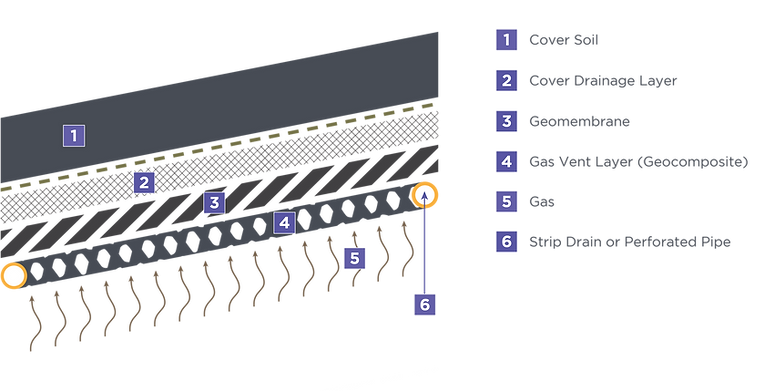# DESIGN METHODS & CONCEPTS

Landfill gases are generated from the biodegradation of solid waste in a landfill. The actual rate of gas generation depends on waste composition, moisture content, age, etc. The purpose of a gas collection layer is to facilitate the collection of the generated gases so that they do not cause uplift of the cap. The typical configuration of a landfill gas collection layer is presented in Figure 7.1. The primary design criterion for geocomposites is to provide enough flow capacity to reduce the landfill gas pressure to an acceptable level in terms of factor of safety for slope stability, as illustrated in the following equation:

# s                cover                cover

EQUATION 7.1

WHERE

u        = allowable gas pressure (kPa)
γ         = cover soil density (kg/ m  )

t          = soil cover thickness (m)
FS   = factor of safety against sliding
δ = interface friction angle (degrees) for geocomposite-geomembrane interface
β = slope angle

# 3FIGURE 7.1 – SCHEMATIC OF A LANDFILL GAS COLLECTION LAYER

The incoming flow rate for landfill gas will be gauged in terms of flux. The equation used to calculate the landfill gas flux is presented as follows [Thiel, 1998]:

EQUATION 7.2

WHERE

# 3

q    = landfill gas supply rate (m/sec)
r    = landfill gas generation rate (m  /sec/kg of waste)
t          = thickness of waste (m);
γ           = unit weight of waste (kg/m  ).

# 3

The required transmissivity of the gas drainage layer can be calculated as follows:

EQUATION 7.3

WHERE

# 3

q    = landfill gas supply rate (m/sec)
γ   = unit weight of gas (kg/m  )

θ         = required gas transmissivity for geonet or geocomposite (m  /sec per m width)

D = slope distance between drains (m)

# 3

Ultimate gas transmissivity can be calculated using Equation 7.4

EQUATION 7.4

WHERE

# ultimate

θ              = ultimate gas transmissivity
θ      = the transmissivity of the geocomposite (m  /sec-m)

FS = overall factor of safety

RF     = reduction factor for intrusion

RF     = reduction factor for creep to account for long-term behavior

RF     = reduction factor for chemical clogging

RF     = reduction factor for biological clogging

# 2

Notice that the above equation provides the required transmissivity for the flow of gas, not water. Therefore, transmissivity value from actual in-plan airflow should be used for evaluating geocomposite performance.

Table 1 provides density and viscosity values for various fluids for use in Equation 7.5. Again we note that a very significant side benefit of providing a gas collection layer under the final cover is that it will also serve to collect side slope seeps. The seeps would be collected at the toe of the geocomposite gas collection layer, as illustrated in Figure 7.2.

## 1.28E+01

Notes:
( 1 ) Values for LFG (landfill gas) were assumed to be prorated as 55% properties of carbon dioxide, and 45% properties of methane. This ratio was used to match the LFG characteristics for the Coffin Butte case history, which may be different than other landfills.

( 2 ) Values are at standard temperature and pressure.

## KINEMATIC VISCOSITY (ν=μ/ρ)FIGURE 7.2 SEEP COLLECTION AT TOE OF GAS COLLECTION LAYER UNDER FINAL COVER SYSTEM

LFG pressure gradient varies linearly with its maximum at the strip drain location, and zero in the center of the geocomposite gas venting blanket.

Maximum pressure gradient is shown below in equation 7.5:

Maximum pressure gradient is shown in equation 7.5:

# )

EQUATION 7.5

WHERE

q    = landfill gas supply rate (m/sec)

θ         = required gas transmissivity for geonet or geocomposite (m  /sec per m width)

D = slope distance between drains (m)

# EQUATION SHEET

## Required Air Transmissivity and Gradient

Solve For Maximum Gas Pressure

Input parameters

SOLUTION

## tanδ

Solve For Landfill Gas Supply Rate

Input parameters

SOLUTION

## Landfill gass supply rate

Solve For Required Air Transmissivity and Gradient

Input Parameter

SOLUTION